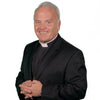### We ask you, humbly, to help.Hi readers, it seems you use Catholic Online School a lot; that's great! It's a little awkward to ask, but we need your help. If you have already donated, we sincerely thank you. We're not salespeople, but we depend on donations averaging \$14.76 and fewer than 1% of readers give. If you donate just \$5.00, the price of your coffee, Catholic Online School could keep thriving. Thank you. Help Now >

# MATHEMATICS

Algebra I - Students will study the basic concepts of Algebra. The course will teach: evaluation of expressions involving signed numbers and the absolute value of numbers; exponents and roots; properties of real numbers; solving linear and simultaneous equations; polynomials and rational expressions; word problems; the Pythagorean theorem; algebraic proofs, functions, and functional notation; scientific notation; solution of quadratic equations; multiplication and division of polynomials; domain and range; unit conversions; computation of perimeter, area, surface area, and volume; and statistics and probability.

Algebra II - This course will teach advanced concepts in Algebra as well as some basic geometry. Topics include: imaginary and complex roots of quadratic equations; solutions of simultaneous linear and non-linear equations and inequalities; conic sections; logarithms and antilogarithms; exponential equations; basic trigonometric functions; advanced factoring techniques; proofs of congruence; deductive reasoning; properties of parallel and perpendicular lines; properties of triangles; chords, arcs, and inscribed angles in circles; basic geometric constructions; Venn diagrams; vectors; polar and rectangular coordinate systems; set theory; advanced algebraic word problems; and mathematical aspects related to concepts in physics and chemistry.

Geometry - Students will study classical geometry. Concepts include: points, lines, planes, and angles; deductive reasoning; parallel lines and planes; congruent triangles; quadrilaterals; inequalities, similar polygons; right triangles; circles; construction and loci; areas of plane figures; and areas and volumes of solids. Students will also learn proofs and real-world applications of geometry.

Advanced Math I - Students will study geometry, trigonometry, and advanced algebra in this blended course. Students will study: reciprocal, exponential, combining, and decomposing functions; sums of and inverse trigonometric functions; solving trigonometric equations using factoring; laws of logarithms and logarithmic equations; linear regression; permutations; factoring over the complex plane; abstract coefficients; single variable analysis; and polar forms of complex numbers.

Advanced Math II - Students will continue their study of mathematics though this course which introduces: radicals in trigonometric equations; locus concepts in analytic geometry; matrices, matrix algebra, and inverse matrices; determinants and solutions of equations using determinants; law of sines and law of cosines; combinations; trigonometric identities; De Moivre’s Theorem; geometric progressions and series; arithmetic and geometric means; logarithmic inequalities; binomial theorem; synthetic division; the Remainder Theorem; and solving solutions of equations using a graphing calculator.

Calculus - This class is intended for those who seek a career in that requires robust math skills, such as work in physics, engineering, business, or the life sciences. Students will learn: the derivative; the derivative of sums and differences; the derivatives of a quotient; implicit differentiation; derivatives of inverse functions; the derivatives of specific functions including logarithmic and trigonometric functions; the differential; product rule for derivatives and differentials; the chain rule; differential equations; integration; the integrals of specific functions including logarithmic and trigonometric functions; and integration by parts.

Consumer Math - In this course, students will learn practical math skills, such as budgeting, calculating interest, buying a car, buying a home, doing taxes, and other challenges. Problems are presented in a practical, real-world format so students are prepared to use their skills upon completion.

Logic - Logic teaches the rules and concepts of logic and reasoning. Students will learn how to recognize fallacies, how to state arguments in logical form, and how to challenge and defend claims.